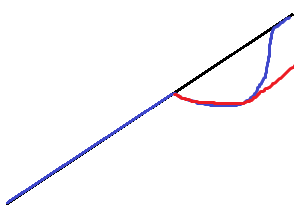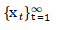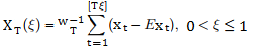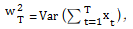# Order of Integration (Time Series): Simple Definition / Overview

Share on

“Order of integration” is a summary statistic used to describe a unit root process in time series analysis. Specifically, it tells you the minimum number of differences needed to get a stationary series.

## The Problem of Unit RootsA possible unit root. The red line shows the drop in output and path of recovery if the time series has a unit root. Blue shows the recovery if there is no unit root and the series is trend-stationary.

An ideal time series has stationarity. That means that a shift in time doesn’t cause a change in the shape of the distribution. Unit root processes are one cause for non-stationarity.

## Order of Integration I(d)

If you have unit roots in your time series, a series of successive differences, d, can transform the time series into one with stationarity. The differences are denoted by I(d), where d is the order of integration. Non-stationary time series that can be transformed in this way are called series integrated of order k. Usually, the order of integration is either I(0) or I(1); It’s rare to see values for d that are 2 or more.

## So, what exactly is an I(0) process?

An I(0) process is a non-integrated (stationary) process. Unfortunately, there’s no consensus on what exactly constitutes an integrated process, and what constitutes a non-integrated one. Burke & Hunter (2005) suggest that one way to view order of integration is “…being the number of unit roots in the autoregressive lag polynomial”.
Davidson, his paper, When is a Time Series I(0)?, states that “Since the inception of integrated time series modelling in econometrics, the question of what constitutes a non-integrated process has remained troublingly elusive.” He goes on to list no fewer than 5 different definitions from various sources, including:

• “A series with no deterministic component which has a stationary, invertible ARMA representation after differencing d times is said to be integrated of order d…(Engle and Granger 1987, p. 252.)”
• and

• A finite (non-zero) variance stochastic process which does not accumulate past errors is said to be integrated of order zero…(Hendry 1995, p. 43).”

Davidson suggests that it may be easier think of an I(0) process as specific conditions that make asymptotic theory valid. That said, this simple definition does require some understanding of not-so-simple processes (like the concept of a limit in calculus and asymptotic theory). The definition is as follows:
A time seriesis I(0) if the partial sum process XT, defined on the unit interval bywhereconverges weakly to standard Brownian motion B as T → 1.

## How to Find the Integration Order

The first step in transforming a time series is to see if you have a unit root process in the first place. Sometimes, a simple plot of the data will reveal the presence of a unit root (as in the image at the top of this article). However, looking at a plot — even one that obviously has a unit root — doesn’t give you the integration order. For that, you’ll have to perform further analysis. Two popular options are the KPSS test and the Augmented Dickey-Fuller test. For the AGF test, Dickey & Pantula (1987) suggest running the test assuming a higher order of integration (say, I(2))and working downwards to avoid the problem of over-differencing.

References:
Burke & Hunter. (2005). Modelling Non-Stationary Economic Time Series: A Multivariate Approach.
Davidson, J. When is a Time Series I(0)? In The methodology and practice of econometrics : a festschrift in honour of David F. Hendry. Oxford ; New York : Oxford University Press, 2009. Retrieved 8/13/2017 from: http://people.exeter.ac.uk/jehd201/WhenisI0.pdf
Dickey,D. & Pantula, S. (1987) Determining the order of differencing in autoregressive processes. Journal of Business and Economic Statistics. 5(4). 455-461.
Engle, R. F. and Granger, C. W. J. (1991) Long-run Economic Relationships: Readings in Cointegration, Oxford University Press.
Hendry, D. F. (1995) Dynamic Econometrics. Oxford: Oxford University Press.

CITE THIS AS:
Stephanie Glen. "Order of Integration (Time Series): Simple Definition / Overview" From StatisticsHowTo.com: Elementary Statistics for the rest of us! https://www.statisticshowto.com/order-of-integration/
---------------------------------------------------------------------------Need help with a homework or test question? With Chegg Study, you can get step-by-step solutions to your questions from an expert in the field. Your first 30 minutes with a Chegg tutor is free!